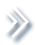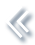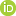####ArchivesReview Articles

## Rates of convergence of powered order statistics from general error distribution

Yuhan Zou ,

School of Mathematics and Statistics, Southwest University, Chongqing, People's Republic of ChinaYingyin Lu ,

School of Science, Southwest Petroleum University, Chengdu, People's Republic of ChinaZuoxiang Peng

School of Mathematics and Statistics, Southwest University, Chongqing, People's Republic of Chinapzx@swu.edu.cn

Pages | Received 03 May. 2022, Accepted 04 Nov. 2022, Published online: 21 Nov. 2022,
• Abstract
• References
• Citations

Let {Xn,n≥1}{Xn,n≥1} be a sequence of independent random variables with common general error distribution GED(v)GED(v) with shape parameter v>0, and let Mn,rMn,r denote the rth largest order statistics of X1,X2,…,XnX1,X2,…,Xn. With different normalizing constants the distributional expansions and the uniform convergence rates of normalized powered order statistics |Mn,r|p|Mn,r|p are established. An alternative method is presented to estimate the probability of the rth extremes. Numerical analyses are provided to support the main results.

• Cao, W., & Zhang, Z. (2021). New extreme value theory for maxima of maxima. Statistical Theory and Related Fields5(3), 232–252. https://doi.org/10.1080/24754269.2020.1846115
• Hall, P. (1979). On the rate of convergence of normal extremes. Journal of Applied Probability16(2), 433–439. https://doi.org/10.2307/3212912
• Hall, P. (1980). Estimating probabilities for normal extremes. Advances in Applied Probability12(2), 491–500. https://doi.org/10.2307/1426608
• Hashorva, E., Peng, Z., & Weng, Z. (2016). Higher-order expansions of distributions of maxima in a Hüsler-Reiss model. Methodology and Computing in Applied Probability18(1), 181–196. https://doi.org/10.1007/s11009-014-9407-6
• Jia, P., & Li, T. (2014). Higher-order expansions for distributions of extremes from general error distribution. Journal of Inequalities and Applications2014, 213. https://doi.org/10.1186/1029-242X-2014-213
• Leadbetter, M. R., Lindgren, G., & Rootzén, H. (1983). Extremes and related properties of random sequences and processes. Springer Verlag.
• Li, T., & Peng, Z. (2018). Moment convergence of powered normal extremes. Communication in Statistics-Theory and Methods47(14), 3453–3463. https://doi.org/10.1080/03610926.2017.1359294
• Liao, X., Peng, L., Peng, Z., & Zheng, Y. (2016). Dynamic bivariate normal copula. Science China Mathematics59(5), 955–976. https://doi.org/10.1007/s11425-015-5114-1
• Liao, X., & Peng, Z. (2012). Convergence rates of limit distribution of maxima of lognormal samples. Journal of Mathematical Analysis and Applications395(2), 643–653. https://doi.org/10.1016/j.jmaa.2012.05.077
• Liao, X., & Peng, Z. (2014). Convergence rate of maxima of bivariate gaussian arrays to the Hüsler–Reiss distribution. Statistics and Its Interface7(3), 351–362. https://doi.org/10.4310/SII.2014.v7.n3.a5
• Liao, X., & Peng, Z. (2015). Asymptotics for the maxima and minima of Hüsler–Reiss bivariate gaussian arrays. Extremes18, 1–14. https://doi.org/10.1007/s10687-014-0196-7
• Liao, X., Peng, Z., & Nadarajah, S. (2014a). Tail behavior and limit distribution of maximum of logarithmic general error distribution. Communications in Statistics-Theory and Methods43(24), 5276–5289. https://doi.org/10.1080/03610926.2012.730168
• Liao, X., Peng, Z., Nadarajah, S., & Wang, X. (2014b, January). Rates of convergence of extremes from skew-normal samples. Statistics and Probability Letters84, 40–47. https://doi.org/10.1016/j.spl.2013.09.027
• Lu, Y., & Peng, Z. (2017). Maxima and minima of independent and non-identically distributed bivariate Gaussian triangular arrays. Extremes20, 187–198. https://doi.org/10.1007/s10687-016-0263-3
• Nair, K. A. (1981). Asymptotic distribution and moments of normal extremes. Annals of Probability9(1), 150–153. https://doi.org/10.1214/aop/1176994515
• Nelson, D. B. (1991). Conditional heteroskedasticity in asset returns: A new approach. Econometrica59(2), 347–370. https://doi.org/10.2307/2938260
• Peng, Z., Nadarajah, S., & Lin, F. (2010). Convergence rate of extremes for the general error distribution. Journal of Applied Probability47(3), 668–679. https://doi.org/10.1239/jap/1285335402
• Peng, Z., Tong, B., & Nadarajah, S. (2009). Tail behavior of the general error distribution. Communications in Statistics-Thoery and Methods38(11), 1884–1892. https://doi.org/10.1080/03610920802478367
• Resnick, S. I. (1987). Extreme values, regular variation and point processes. Springer-Verlag.
• Zhang, Z. (2021). On studying extreme values and systematic risks with nonlinear time series models and tail dependence measures. Statistical Theory and Related Fields5(1), 1–25. https://doi.org/10.1080/24754269.2020.1856590
• Zhou, W., & Ling, C. (2016, April). Higher-order expansions of powered extremes of normal samples. Statistics and Probability Letters111, 12–17. https://doi.org/10.1016/j.spl.2016.01.003

To cite this article: Yuhan Zou, Yingyin Lu & Zuoxiang Peng (2023) Rates of convergence of powered order statistics from general error distribution, Statistical Theory and Related Fields, 7:1, 1-29, DOI: 10.1080/24754269.2022.2146955 To link to this article: https://doi.org/10.1080/24754269.2022.2146955